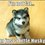# Help needed!

1.Let $\alpha$ and $\beta$ be the two roots of the cubic polynomial $x^3+ax^2+bx+c$ satisfying $\alpha\beta+1 = 0$.Prove that $c^2+ac+b+1=0$ .

2.Solve for real $x$: $x^{\sqrt{x}}=\sqrt{x^x}$

3.If polynomial $p(x) = Ax^3+Bx^2+Cx+D$ vanishes at $x=a-d,a,a+d$ then prove that $a^2+\frac{D}{aA}>0$.Here $A,B,C ,D$ are constants.

Please provide a solution to these problems as soon as possible.Thanks.Note by Anik Mandal
5 years, 1 month ago

This discussion board is a place to discuss our Daily Challenges and the math and science related to those challenges. Explanations are more than just a solution — they should explain the steps and thinking strategies that you used to obtain the solution. Comments should further the discussion of math and science.

When posting on Brilliant:

• Use the emojis to react to an explanation, whether you're congratulating a job well done , or just really confused .
• Ask specific questions about the challenge or the steps in somebody's explanation. Well-posed questions can add a lot to the discussion, but posting "I don't understand!" doesn't help anyone.
• Try to contribute something new to the discussion, whether it is an extension, generalization or other idea related to the challenge.
• Stay on topic — we're all here to learn more about math and science, not to hear about your favorite get-rich-quick scheme or current world events.

MarkdownAppears as
*italics* or _italics_ italics
**bold** or __bold__ bold
- bulleted- list
• bulleted
• list
1. numbered2. list
1. numbered
2. list
Note: you must add a full line of space before and after lists for them to show up correctly
paragraph 1paragraph 2

paragraph 1

paragraph 2

[example link](https://brilliant.org)example link
> This is a quote
This is a quote
    # I indented these lines
# 4 spaces, and now they show
# up as a code block.

print "hello world"
# I indented these lines
# 4 spaces, and now they show
# up as a code block.

print "hello world"
MathAppears as
Remember to wrap math in $$ ... $$ or $ ... $ to ensure proper formatting.
2 \times 3 $2 \times 3$
2^{34} $2^{34}$
a_{i-1} $a_{i-1}$
\frac{2}{3} $\frac{2}{3}$
\sqrt{2} $\sqrt{2}$
\sum_{i=1}^3 $\sum_{i=1}^3$
\sin \theta $\sin \theta$
\boxed{123} $\boxed{123}$

## Comments

Sort by:

Top Newest

$1.$ Let the third root be $\gamma$. $\alpha\beta=-1\implies \alpha\beta\gamma=-\gamma.....(I)$ By Vieta's: $\alpha\beta\gamma=-c$. Substituting in $I$ to get $\gamma=c$ and since $\gamma$ is a root of cubic equation:- $c^3+ac^2+bc+c=0\implies c^2+ac+b+1=0$

$2.$ We always look for $0,1$ in these equations. Obviously $x=1$ is a solution. Now, $\Large{x^{\sqrt x}=x^{x/2}}$ $\Large\implies \sqrt x=x/2$ $\Large \implies \sqrt x(\sqrt x-2)=0$ $\Large \implies \sqrt x=2\implies x=4$ ($x=0$ is obviously rejected due to indeterminate form)

Combining $x=1,4$.

$3.$ By Vieta's $-D/A=(a-d)a(a+d)$$\implies \dfrac{-D}{aA}=(a-d)(a+d)=a^2-d^2$. $\implies a^2+\dfrac{D}{aA}=a^2+(d^2-a^2)=d^2>0~~(d\neq 0)$.

- 5 years, 1 month ago

Log in to reply

In the third question,there is a small typo.It should be $a^2+\frac{D}{aA}$

- 5 years, 1 month ago

Log in to reply

Thanks .... corrected.

- 5 years, 1 month ago

Log in to reply

Thanks a lot for your reply!

- 5 years, 1 month ago

Log in to reply

What have you tried?

Staff - 5 years, 1 month ago

Log in to reply

I had tried using Vieta's and I have now got the solutions.

- 5 years, 1 month ago

Log in to reply

×

Problem Loading...

Note Loading...

Set Loading...